# Conditional Probability

The conditional probability of an event B is the probability that the event will occur given that an event A has already occurred.

Probability Notation: P(B | A) is read as the probability of event B given that event A happened.

We can express the conditional probability of an event like this: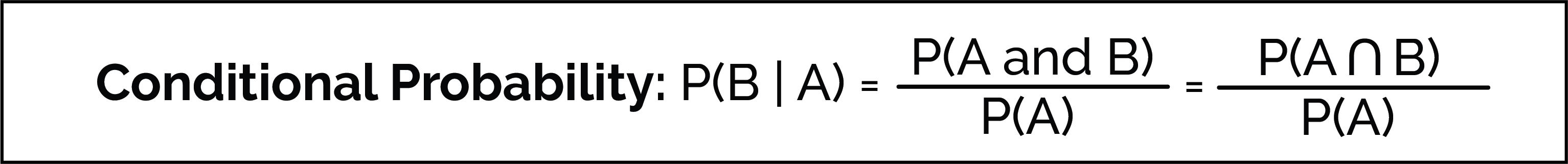• This expression is only valid when P(A) is not equal to 0.
• In the case where events A and B are independent (when event A has no effect on the probability of event B), the conditional probability of event B given A is just the probability of event B: P(B).

One way to solve problems with 2 or more conditions is to use tables.

Example: Genetic Testing
A genetic test has been developed to detect if you are related to Taylor Swift. Experimentally, scientists have found the following statistics about the test:

1. Among all people, 1% are related to Taylor Swift.
2. If you are related to Taylor Swift, you have a 99% chance to get a positive test result: (+). (“True positive”)
3. If you are not related to Taylor Swift, you have only a 6% chance to get a positive test result: (+). (“False positive”)
• This means that 94% of the time you get a “true negative” result”

Let’s look at a group of 200 people to answer the following question:
Given that you got a positive test results, what’s the probability you are actually related to Taylor Swift?

Solution: Contingency Table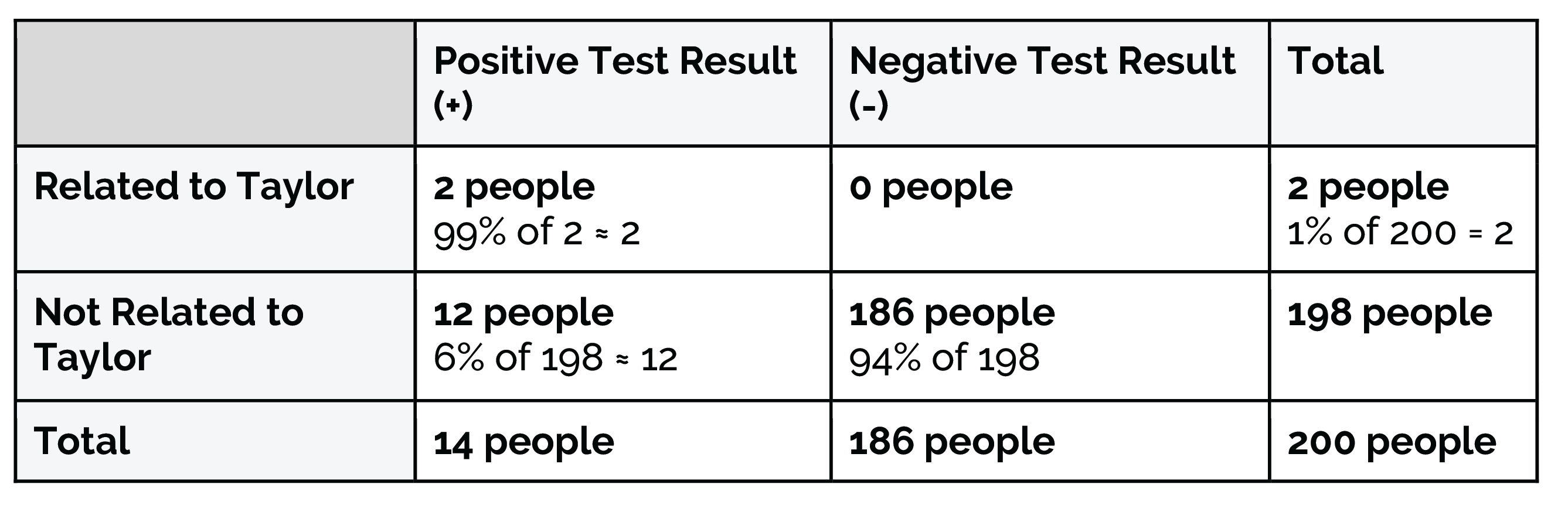If we fill out the contingency table with the information given, we can see that there were 14 total people who got positive results. Out of those 14 people, only 2 of them were actually related to Taylor Swift!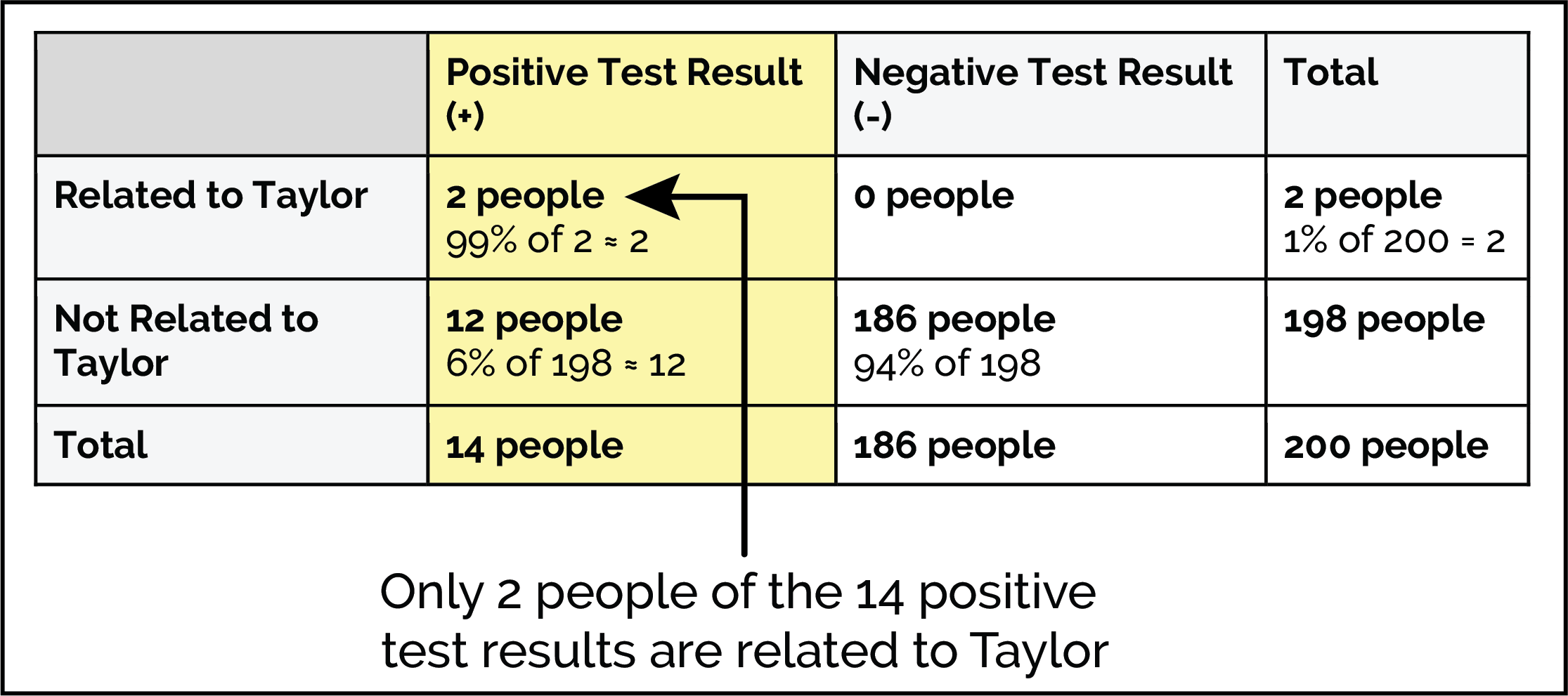So the answer to our question is about 2/14 or 1/7 = ~14%.

Contingency Tables are one way to solve conditional probability problems. There are other ways as well, such as Baye’s Rule (link to the Baye’s Rule page).

# Example Walk-Throughs with Worksheets

### Video 1: Conditional Probability Examples

Follow along with the workseet to work through the problem:

# Practice Questions

Q1: In which case would "Here" print in the following if-statement?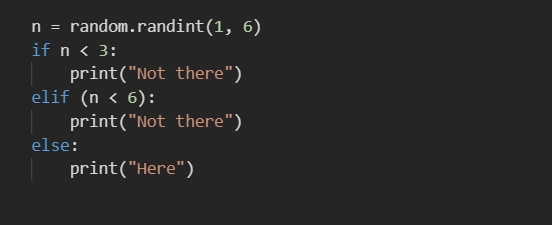Q2: Which of the following is not part of the correct syntax for an if-statement?
Q3: What would the value of n be after running the follwing code chunk?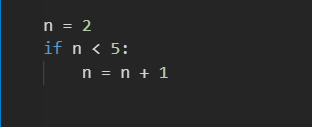Q4: What would print after running the follwing code chunk?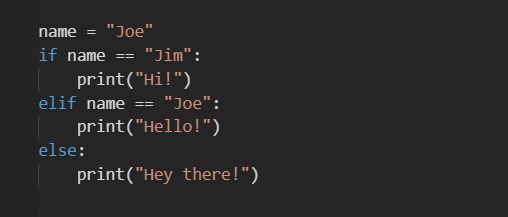Q5: What would print after running the following code chunk?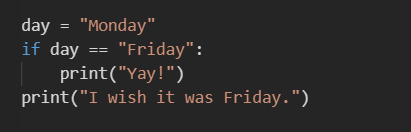Q6: In a normal distribution, which two statistics are both equal to zero?